Courses

Revision Notes: Ray Optics Notes | Study NEET Revision Notes - JEE

JEE: Revision Notes: Ray Optics Notes | Study NEET Revision Notes - JEE

The document Revision Notes: Ray Optics Notes | Study NEET Revision Notes - JEE is a part of the JEE Course NEET Revision Notes.
All you need of JEE at this link: JEE

Basic Terms Used in Ray Optics

• Light: It is an agent which produces in us the sensation of sight. It is a form of energy.
• Transparent Medium: It is a medium through which light can be propagated easily.
Examples: Sun, Candle, Electric Arc.
• Translucent Medium: It is a medium through which light is propagated partially.
Examples: Paper, Ground, Glass.
• Opaque: It is a medium through which light cannot be propagated.
Examples: Wood, Iron.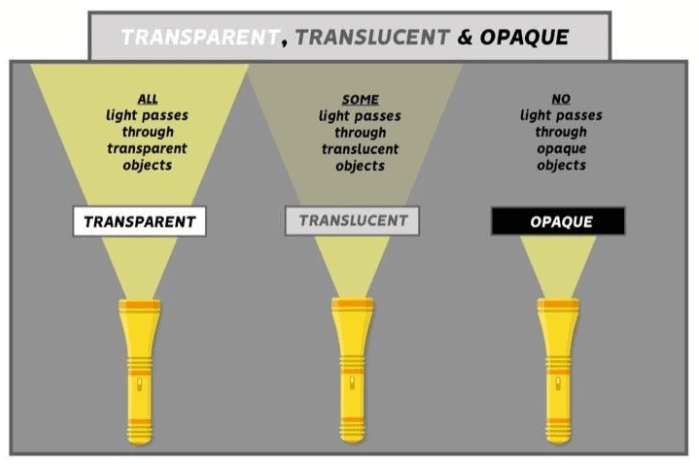What is Reflection of Light?

It is the property of light by virtue of which, light is sent back into the same medium from which it is coming after being obstructed by a surface.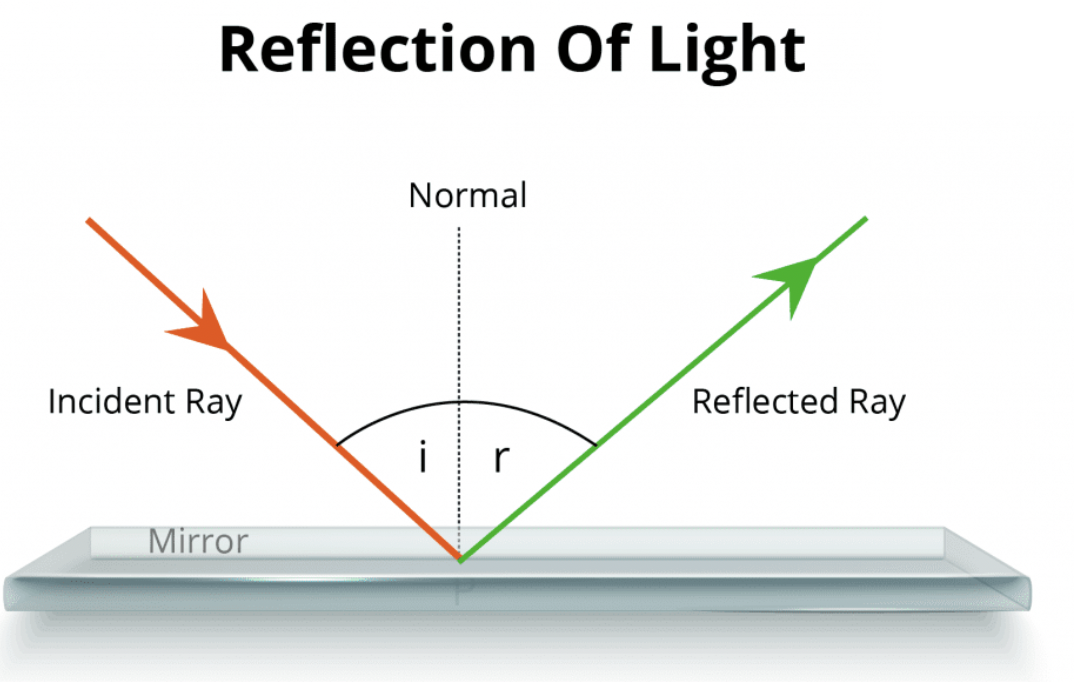Laws of Reflection

1. The incident ray, the reflected ray, and normal to the reflecting surface at the point of incidence, all lie in one plane and that plane is perpendicular to the reflecting surface.
2. The angle of incidence is equal to the angle of reflection.
So, ∠i = ∠r

Reflection from a plane mirror

When an object is placed in front of a plane mirror, its image can be seen behind the mirror. The distance of the object from the mirror is equal to the distance of the image from the mirror.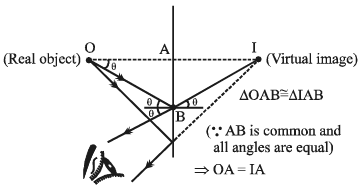1. The magnification of a plane mirror is unity.
2. The image is formed behind the mirror. It is erect. Virtual and laterally inverted.
3. Image formation by two inclined mirrors, inclined at angle = θ∈[0, 180°]
4. The object and all its images will always lie on a circle, having center at the point of intersection of the two inclined mirrors, in a two dimensional view.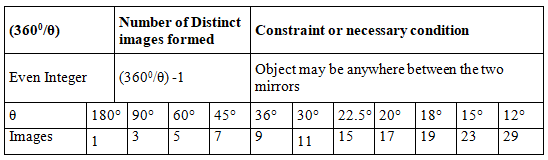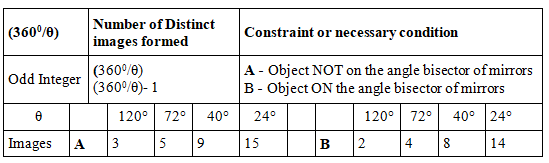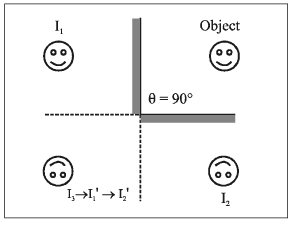Other Important Information

• When the object moves with speed u towards (or away) from the plane mirror then the image also moves toward (or away) with speed u. But the relative speed of image w.r.t. object is 2u.
• When the mirror moves towards the stationary object with speed u, the image will move with speed 2u.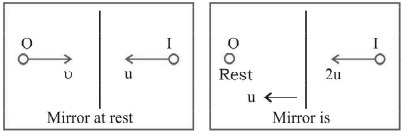Concave and Convex Mirror

• Concave Mirror: It is a spherical mirror which when looked at from the reflecting side is depressed at the centre and bulging at the edges.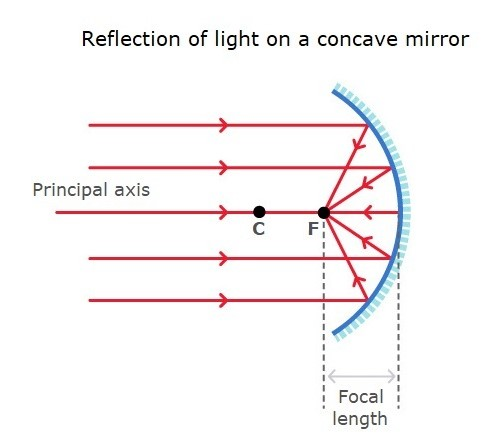• Convex Mirror: It is a spherical mirror which when looked at from the reflecting side bulges at the centre and is depressed at the edges.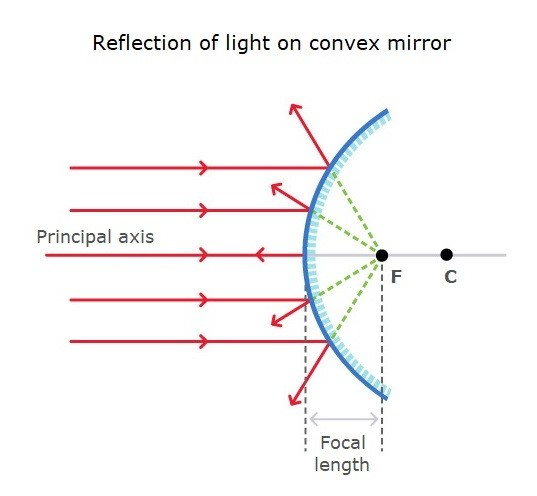Important Terms

• Radius of Curvature(R): The radius curvature of a mirror is defined as the radius of that sphere of which the mirror forms a part.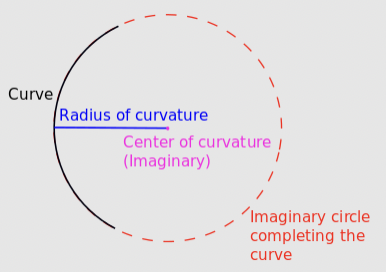• Principal Focus: Principal focus is a point, situated on the principal axis, at which a beam coming parallel to the principal axis meets or appears to meet after reflection from the mirror.
• Focal Plane: It is a vertical plane passing through the principal focus and perpendicular to the principal axis.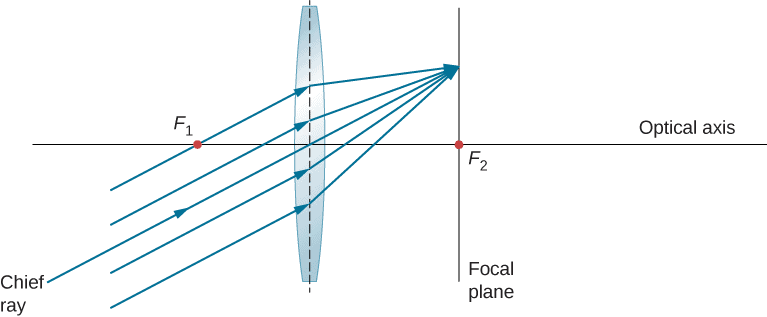• Focal Length(f): Focal length, of a spherical mirror, is the distance of its principal focus from its pole.
• Relation between focal length and radius of curvature

f = R/2

This signifies that the focal length of a spherical mirror is half of its radius of curvature.

• Mirror formula

1/f = 1/v + 1/u = 2/r

• Relative positions, size and nature of the image as an object is brought from infinity to the pole of a concave mirror
(a) If the object is at infinity,
u = ∞, v = -f, m = 0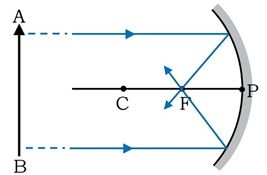Thus, the image is obtained at the focus of a focal plane and very small in dimensions.
(b) If object lies beyond centre of curvature,
2f > v > f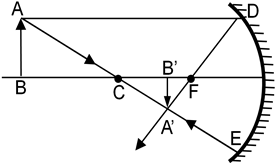The image thus formed is diminished, real and inverted.
(c) If object is at centre of curvature,
v = -2f , m =1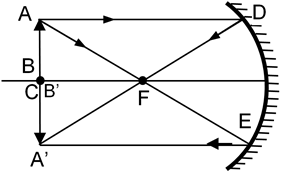Thus, a real and inverted image of same size as that of object is formed at centre of curvature.
(d) Object is in between a distance f and 2f, i.e., in between focus and centre of curvature,
(f < u < 2f), v < ∞ and m = (v/u) >1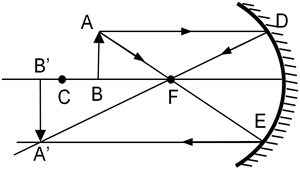Thus, a real, inverted and magnified image is formed in between centre of curvature and infinity.
(e) Object is kept at focus,
(u = -f), v = -∞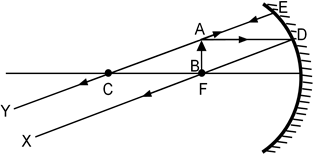The rays after reflection are rendered into a parallel beam meeting in infinity.
(f) Object is kept within focus (u < -f),
v is positive.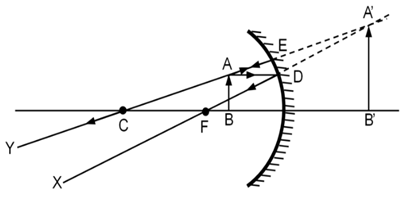Thus, a virtual, erect and magnified image is formed on the other side of the mirror.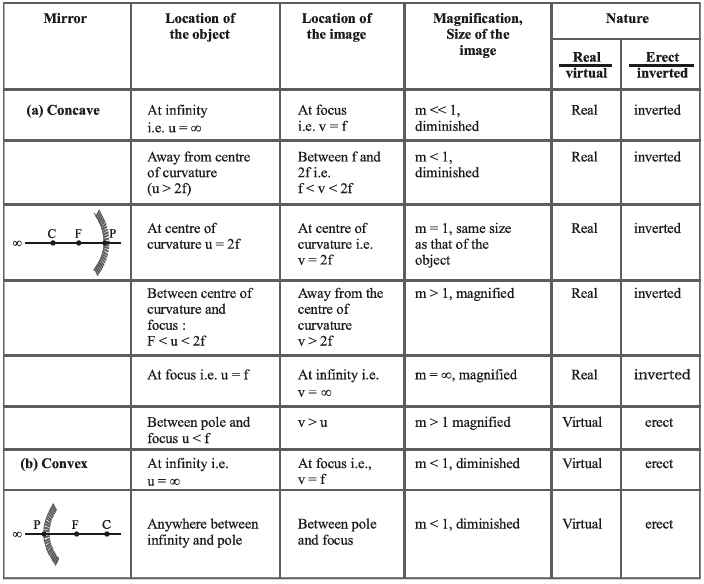Use the following sign while solving the problem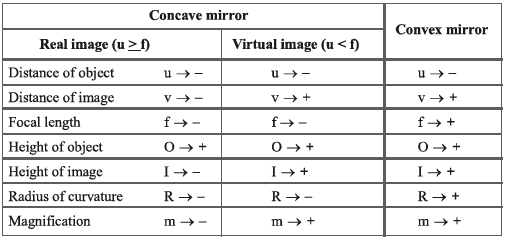Some Important Points:

• In the case of spherical mirrors, the focal length is half its radius of curvature image.
• In the case of the concave mirror, a real object produces a real and inverted if its distance from the pole is greater than the focal length while its virtual and erect if its distance from the pole is less than the focal length.
• In the case of a convex mirror, a real object always produces a virtual and erect image.
• All real images are inverted and virtual images are erect.
•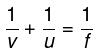•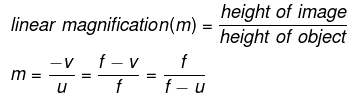• Keep in mind the sign conventions and substitute each value with the sign. This makes a formula suitable to be applied in any case.

Try yourself:A convex mirror is used to form an image of a real object. Then mark the wrong statement

What is Refraction?

Refraction is the phenomenon by virtue of which a ray of light going from one medium to the other undergoes a change in its velocity.

• Incident ray: The ray which approaches the interface is called an incident ray.
• Refracted ray: Ray which goes into the second medium is called refracted ray.
• Laws of Refraction
(a) Snell’s law: The sine of the angle of incidence bears a constant ratio with the sine of the angle of refraction.
i.e. sin i / sin r = constant
(b) The incident ray, the refracted ray and the normal to the interface at the point of incidence all lie in one plane and that plane is perpendicular to the interface separating the two media.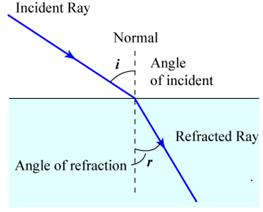• Refractive Index
(a) Refractive index of a medium with respect to another is defined as the ratio between the sine of the angle of incidence to the sine of the angle of refraction.
i.e. sin i / sin r = constant = 1µ2
(b) Refractive index of medium 2 with respect to 1 is also defined as the ratio between the velocity of light in medium 1 to the velocity of light in medium 2.
i.e. 1µ2 = v1/v2
(c) Absolute refractive index of a medium is defined as refractive index of the medium with respect to air, or the ratio between the velocity of light in air to the velocity of light in the medium.
i.e. µ = c/v
(e) Refractive index of a second medium with respect to the first is defined as the ratio between the absolute refractive index of the second medium to the absolute refractive index of the first medium.
i.e. 1µ2 = µ21

Try yourself:When a light ray from a rarer medium is refracted into a denser medium, its

• Single Refraction from a Plane Surface Real and Apparent Depth
When an object placed in a medium is seen from another medium, its apparent position is different from the actual position. Consider the following figure.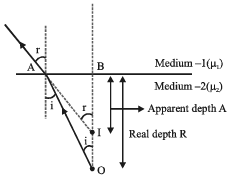We shall derive the expression for small angles (or you can say that the object is being seen from top). By Snell’s law,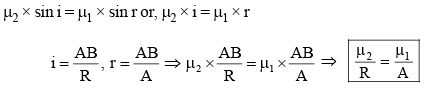The following possibilities may arise.
(i) When observer is in air and the object is in a medium of refractive index μ,
You have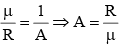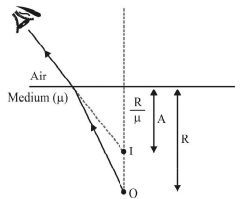(ii) When observer is in a medium of refractive index μ and the object is in air, you have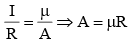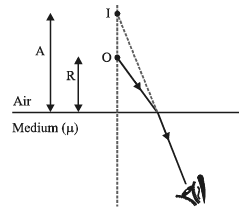• Shift due to a Glass Slab (Double Refraction from Plane Surfaces)
(i) Normal Shift : Here, again two cases are possible.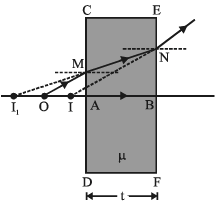An object is placed at O. Plane surface CD forms its image (virtual) at I1. This image acts as object for EF which finally forms the image (virtual) at I. Distance OI is called the normal shift and its value is,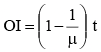This can be proved as under:
Let OA = x then AI1 = μx (Refraction from CD)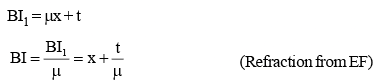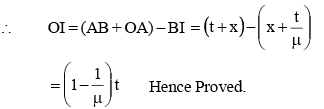(ii) Lateral Shift : We have already discussed that ray MA is parallel to ray BN. But the emergent ray is displaced laterally by a distance d, which depends on , t and i and its value is given by the relation,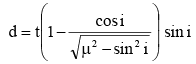• Total Internal Reflection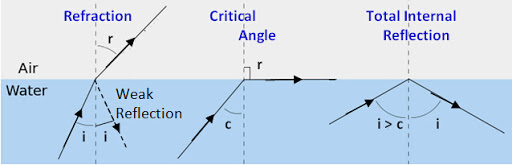(a) Critical angle: Critical angle is the angle of incidence of a ray of light in a denser medium such that its angle of refraction in the rarer medium is 90º.
(b) Total Internal Reflection: It is the phenomenon by virtue of which, a ray of light travelling from a denser to a rarer medium is sent back in the same medium provided, it is incident on the interface at an angle greater than the critical angle.
(c) μ = 1/sin C

Refraction through curved surfaces

• Refraction at a single spherical surface when light travelling from the medium of refractive index μ1 (rarer) to that of refractive index μ2 (denser).
(a) Refraction at a convex surface producing real image:
μ2/v – μ1/u = (μ2 - μ1)/R
(b) Refraction at a convex surface producing virtual image:
μ2/v – μ1/u = (μ2 - μ1)/R
(c) Refraction at a concave surface:
μ2/v – μ1/u = (μ2 - μ1)/R
• Refraction at a single spherical surface when light travelling from the medium of refractive index μ2(denser) to that of refractive index μ1(rarer)
Convex surface producing a real image of a real object:
μ2/u – μ1/v = (μ2 - μ1)/R
• Light travelling from air to glass:
μ/v – 1/u = (μ-1)/R
• Light travelling from glass to air:
μ/u – 1/v = (μ-1)/R
• Linear magnification for spherical refracting surface:
m= (v/μ2) / (u/μ1)

What are Lens?

• Lens: A portion of refracting material bound between two spherical surfaces is called a lens.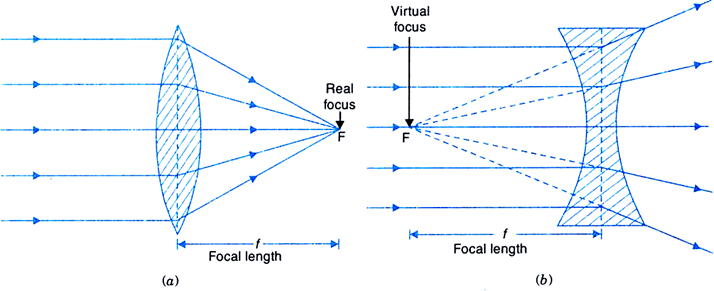(a) Converging lens and (b) Diverging lens
• Converging lens: A lens is said to be converging if the width of the beam decreases after refraction through it.
• Diverging lens: A lens is said to diverge if the width of the beam increases after refraction through it.
• Centre of curvature: Center of curvature of a surface of a lens is defined as the centre of that sphere of which that surface forms a part.
• Radius of curvature: Radius of curvature of a surface of a lens is defined as the radius of that sphere of which the surface forms a part.
• Principal focal length
(a) First principal focal length: First principal focal length of a surface is defined as the distance of that point from the pole of surface from where if a beam diverges or to which a beam converges, the rays after refraction through the surface become parallel to the principal axis.
i.e. f1 = -μ1R/ (μ– μ1)
(b) Second principal focal length: Second principal focal length of a surface is the distance of that point from the pole of the surface at which a beam coming parallel to the principal axis meets or appears to meet after refraction through the surface.
i.e. f2 = μ2R/ (μ2 - μ2)
(c) Relation between f1 and f2: f2/v + f1/u = 1
• Focal length: The distance between optical centre and second principal focus is focal length. Assumptions and sign conventions are same as these of mirrors with optical centre C in place of pole P of the mirror.
• Lens formula: 1/f = 1/v – 1/u
• Lens Maker's formula
1.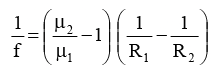2.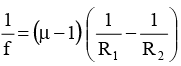3. For a converging lens, R1 is positive and R2 is negative. Therefore,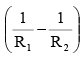in eq. (v) comes out a positive quantity and if the lens is placed in air, (μ – 1) is also a positive quantity. Hence, the focal length f of a converging lens comes out to be positive. For a diverging lens however, R1 is negative and R2 is positive and the focal length f becomes negative.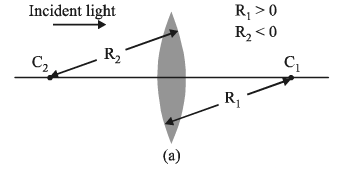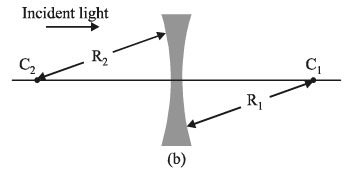4. Focal length of a mirror (fM = R/2) depends only upon the radius of curvature R while that of a lens [eq. (iv)] depends on μ1, μ2, R1 and R2. Thus, if a lens and a mirror are immersed in some liquid, the focal length of lens would change while that of the mirror will remain unchanged.
5. Suppose μ2 < μ1 in eq. (iv), i.e., refractive index of the medium (in which lens is placed) is more than the refractive index of the material of the lens, then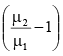becomes a negative quantity, i.e., the lens changes its behaviour. A converging lens behaves as a diverging lens and viceversa. An air bubble in water seems as a convex lens but behaves as a concave (diverging) lens.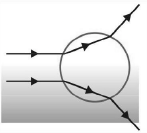• Linear magnification: It is the ratio between the size of the image to the size of the object. i.e. m = I/O
• Expression for m in terms of u, v and f
(a) In terms of v and f: m = [f-v] / f
(b) In terms of u and f: m = f / [f+u]
• Position of the image as the object is gradually moved from infinity to the pole of the lens.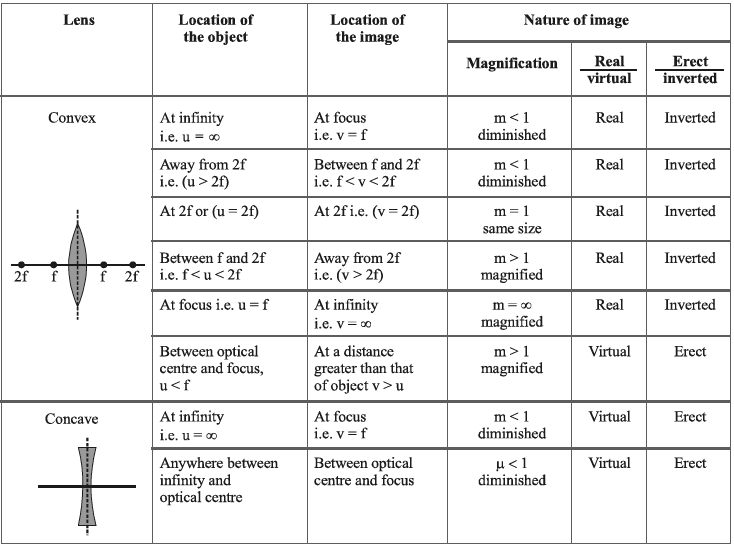Note: Minimum distance between an object and it’s real image formed by a convex lens is 4f. Maximum image distance for concave lens is it’s focal length.

Try yourself:An object is placed at a distance of f / 2 from a convex lens. The image will be

• Refraction through a thin double convex lens when the medium on the two sides of the lens is the same (Lens maker’s formula):
1/f = (μ – 1) (1/R– 1/R2)
• Refraction through a thin double convex lens when the medium situated on the two sides of the lens is different:
1/f = [[μ3 – μ1]/μ3R1] + [[μ3 – μ2]/μ3R2]
• Double concave lens
(a) When the medium situated on the two sides of the lens is the same:
i.e. 1/f = (μ – 1) (1/R– 1/R2)
(b) When the medium situated on the two sides of the lens is different:
i.e. 1/f = [[μ3 – μ1]/μ3R1] + [[μ3 – μ2]/μ3R2]
• Combination of two convex lenses in contact:
F = f1f2 / f1+ f2
• Power of a lens: The reciprocal of the focal length of a lens, expressed in meter, is called its power.
i.e. P = 1/f

What is Prism?

• Refraction through a prism: μ = sin [(A+dm)/2] / sin [A/2]
Here, dm is the minimum angle of deviation.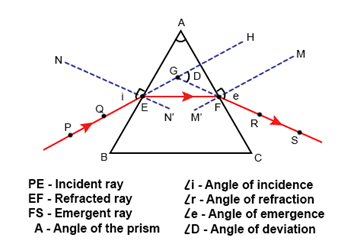Refraction through a Prism
• Refraction through a prism for small angle of incidence:
d = A (μ-1)
This signifies that the angle of deviation d is independent of the angle of incidence, provided it is small.
• Dispersion: The splitting of light into its constituent colors is called dispersion.
• Cauchy’s formula:
μ = A+(B/λ2)+…
Here A and B are constants and λ is the wavelength of light.
• Refraction Through a Prism
(a) Deviation: A ray of monochromatic light (light possessing one wave-length only), while passing through a prism suffers a change in its path, the phenomenon is known as deviation.
i.e. d = (μ-1) A
Here, A is the refractive angle of prism and μ is the refractive index of the material of prism for that particular wavelength of light.
(b) Dispersion: A ray of light (containing more than one wavelengths), while passing through the prism splits up into a number of rays. The phenomenon is called dispersion.
i.e. dv = (μv-1) A and d= (μr-1) A
Here, dv is the deviation for violet and dr is the deviation for red colour. μv and μr be the refractive indices of the material of prism for violet and red colours.
Since, μ> μr, therefore dv is greater than dr.
• Dispersive power (ω): Dispersive power of a prism is defined as the ratio between angular dispersion to mean deviation produced by the prism.
i.e. ω = (dv - dr)/d = (μ– μr)/(μ - 1) = dμ/(μ - 1)
• Spectrum: The band of colours lying side-by-side is called the spectrum.
(a) Impure spectrum: Impure spectrum is a spectrum in which the constituent colours overlap each other.
(b) Pure spectrum: Pure spectrum is a spectrum in which all the constituent colours occupy different and distinct positions.

Try yourself:Yellow light is refracted through a prism producing minimum deviation. If i1 and i2 denote the angle of incidence and emergence for the prism, then

What are Optical Instruments?

• Power of a concave lens(P):
P = (100/x) dioptre,
Here ‘x’ is the distance of a far point of the defective eye, in ‘cm’.
• Magnifying power or magnification of a simple microscope:
M = 1+ (D/f),
Here, ‘D’ is the distance of distinct vision and ‘f’ is the focal length.
• Magnifying power or magnification of a compound microscope:
M = L/f0[1+(D/fe)]
f0 is the focal length of the objective lens, fe is the focal length of the eyepiece and L is the length of the microscope tube.
• Magnifying power or magnification of astronomical telescope (Normal Adjustment):
M = f0/fe
• Magnifying power or magnification of astronomical telescope (When the final image is formed at the distance of distinct vision):
M = (f0/fe) [(fe+D)/D]
• Magnifying power or magnification of Galileo’s telescope:
M = F/f
The document Revision Notes: Ray Optics Notes | Study NEET Revision Notes - JEE is a part of the JEE Course NEET Revision Notes.
All you need of JEE at this link: JEEUse Code STAYHOME200 and get INR 200 additional OFF Use Coupon Code

Top Courses for JEENEET Revision Notes

291 docs

Top Courses for JEETrack your progress, build streaks, highlight & save important lessons and more!

,

,

,

,

,

,

,

,

,

,

,

,

,

,

,

,

,

,

,

,

,

;